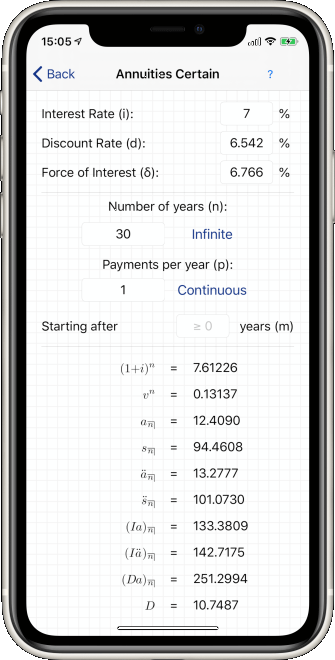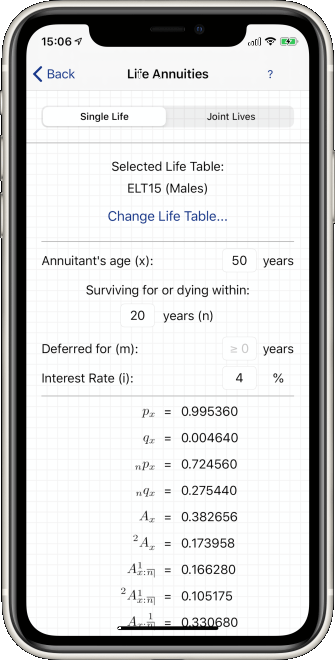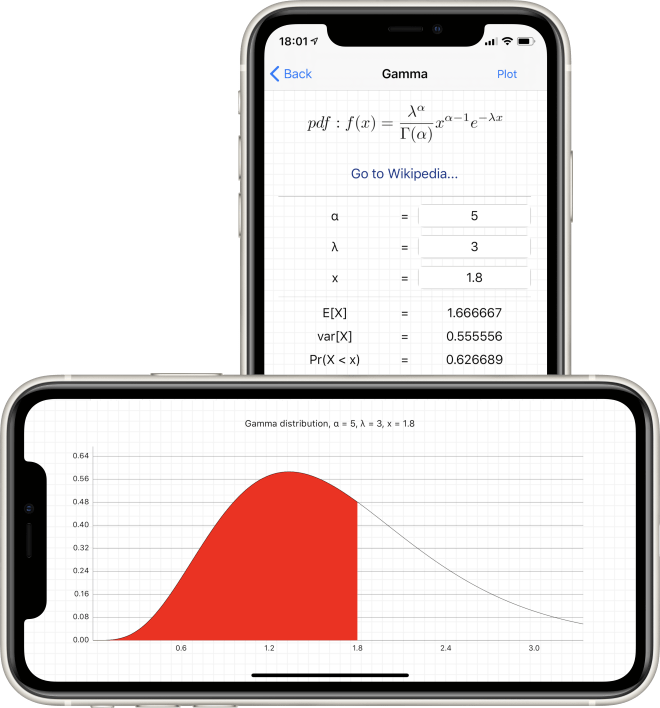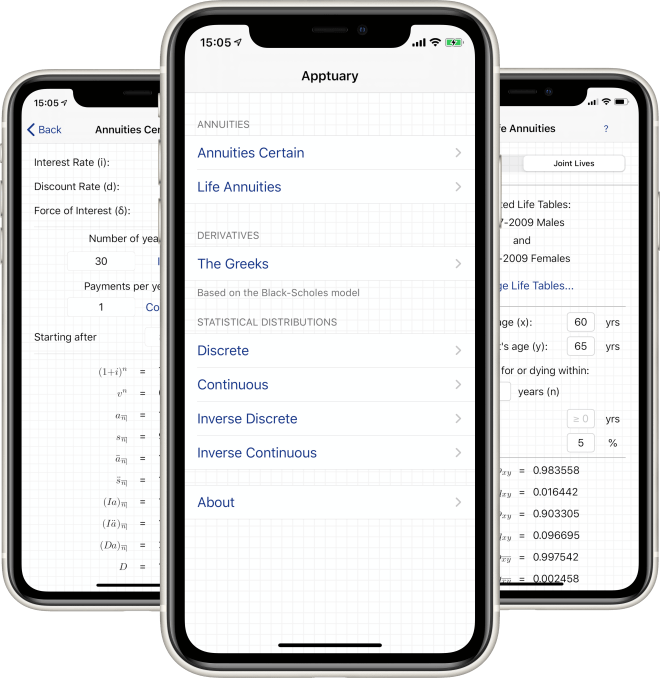"As an Actuarial student, I find this app faster and more extensive than actuarial tables. Great app."

Dave of theresdor (UK)"Great app for most of the distributions Actuaries use. A must-have for an actuary of any level"

ChopsueyCP - USA"As an Actuarial student this is a great little pocket guide to help with the exams! Thanks!"

Gravers - UK"This is a great no-nonsense app for checking my work. Very easy. I wish I could use it on exams!"

Mateo Gcock - USA"A must have for an actuarial student. It can be used to even study while you commute"

Shaikh thaika - UK"Able to solve and show formulas for all of the formulas you may see through exam C. Nice graphical representation as well."

## FEATURES

Apptuary was designed by an Actuary, for other Actuaries and Actuarial students, to help with their day-to-day work and study

#### Any Interest Rate

Unlike pre-calculated compound interest rate tables, Apptuary can calculate statistics using any interest rate you give it

#### Interest Rate, Discount Rate, Force of Interest

Enter any of these to calculate the others, and to calculate the rest of Apptuary's compound interest values

#### Finite or Infinite Payments

AApptuary can calculate values given a number of finite payments, or for infinitely recurring payments#### One or Many Payments Per Year

Want to calculate an annuity that pays out monthly or weekly? Apptuary can calculate it for you!

#### Deferred Payments

Apptuary can calculate the present or future value of a series of payments that starts a number of years away

#### Definitions of Each Symbol

Unsure what a calculated value means? Apptuary contains plain-English definitions of each value it calculates### Fast and Easy Life Insurance Calculations

Simply select a life table and enter an annuitant's age and an interest rate, and you'll have a wide range of calculated values at your fingertips

#### Single and Joint Lives

Easily perform mortality and annuity calculations for both single and joint lives

Apptuary has over 130 different life tables available to use, at the tap of a button

#### Any Interest Rate

Unlike pre-calculated life tables, Apptuary can calculate statistics using any interest rate you give it

#### Definitions of Each Symbol

Unsure what a calculated value means? Apptuary contains plain-English definitions of each value it calculates

#### Discrete Distributions

Calculate moments and probabilities for seven different discrete probability distributions

#### Continuous Distributions

Calculate moments and probabilities for twelve different continuous probability distributions

#### Inverse Distributions

Given a starting probability and a distribution, Apptuary will calculate the inverse for you

#### Distribution Plots

Easily understand the impact of changing parameters by visualising the different distributions